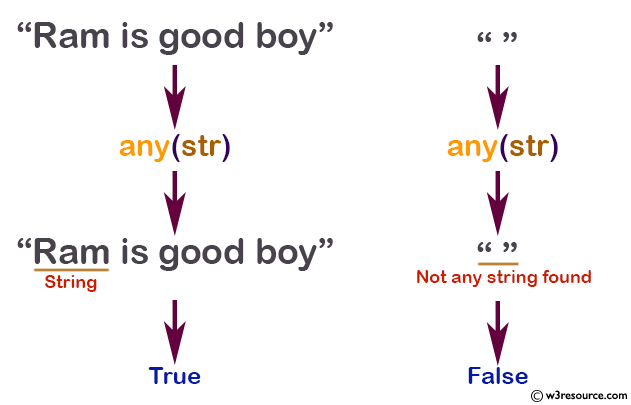# Python: any() function

## any() function

The any() function returns True if any element of the iterable is true. The function returns False if the iterable is empty.

Syntax:

```any(iterable)
```Version:

(Python 3)

Parameter:

Name Description Required / Optional
iterable A container is considered to be iterble, which can return its members sequentially. Required

Return value:

Return True if any element of the iterable is true.

Example:

``````def any(iterable):
for element in iterable:
if element:
return True
return False
``````

Python: any() example with Strings:

``````str = "Ram is good boy"
print(any(str))

# 0 is False
# '0' is True
str = '000'
print(any(str))

str = ''
print(any(str))
``````

Output:

```True
True
False
```

Pictorial Presentation:Python: any() example with Lists:

``````num = [23, 45, 0, 30]
print(any(num)) #True

num = [0, False]
print(any(num)) #False

num = [0, False, 5]
print(any(num)) #True

num = []
print(any(num)) #False
``````

Output:

```True
False
True
False
```

Python: any() example with Dictionaris:

``````dict = {0: 'False'}
print(any(dict))

dict = {0: 'False', 1: 'True'}
print(any(dict))

dict = {0: 'False', False: 0}
print(any(dict))

dict = {}
print(any(dict))

# 0 is False
# '0' is True
dict = {'0': 'False'}
print(any(dict))
``````

Output:

```False
True
False
False
True
```

Python: any() example with Tuple

``````#Test if all items in a tuple are True:
tup = (0, True, False)
x = any(tup)
print(x) #True
tup = (2, 3, 4)
x = any(tup)
print(x) #True
``````

Output:

```True
True
```

Python: any() example with Set

``````#Test if all items in a set are True:
sss = {0, True, False}
x = any(sss)
print(x) #True
sss = (1, True, True)
x = any(sss)
print(x) #True
``````

Output:

```True
True
```

Python Code Editor:

Previous: all()
Next: ascii()

Test your Python skills with w3resource's quiz

﻿

## Python: Tips of the Day

How do I check if a list is empty?

For example, if passed the following:

```a = []
if not a:
print("List is empty")
```

Ref: https://bit.ly/2A4JXx9